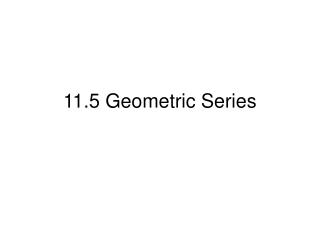DownloadDownload Presentation11.5 Geometric Series

# 11.5 Geometric Series

Download Presentation## 11.5 Geometric Series

- - - - - - - - - - - - - - - - - - - - - - - - - - - E N D - - - - - - - - - - - - - - - - - - - - - - - - - - -
##### Presentation Transcript

1. 11.5 Geometric Series

2. A geometric series is the expression for the sum of the terms of a geometric sequence. Sum of a Finite Geometric Series The sum Sn of a finite geometric series a1 + a2 + a3 + …+ an is

3. Ex. Evaluate the series to the given term. 3+6+12+…; S7 S7 = 3(1-27)/(1-2) = 3(1-128)/-1 = 3(-127)/-1 = 381 the sum of the first 8 terms is 381.

4. In some cases you can evaluate an infinite geometric series. When ‌ r ‌ < 1, the series converges, or gets closer and closer, the sum S. When ‌ r ‌ > or = 1, the series diverges, or approaches no limit. (no sum)

5. Sum of an Infinite Geometric Series An infinite geometric series with ‌ r ‌ < 1 converges to the sum Where a1 is the first term and r is the common ratio.

6. Ex. Give the sum of the infinite geometric series. 1- 1/5 + 1/25 - 1/125 + … Answer: 5/6 HW pg 616 1-17 all